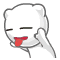Aug 21

#### C++ String的find()成员函数felix021 @ 2008-8-21 14:53 [IT » 程序设计] 评论(2) , 引用(0) , 阅读(10147) | Via 本站原创| |Currently   the   library   contains   only   naive   implementation   of   find   algorithms   with   complexity   O(n   *   m)   where   n   is   the   size   of   the   input   sequence   and   m   is   the   size   of   the   search   sequence.   There   are   algorithms   with   complexity   O(n),   but   for   smaller   sequence   a   constant   overhead   is   rather   big.   For   small   m   < <   n   (m   by   magnitude   smaller   than   n)   the   current   implementation   provides   acceptable   efficiency.   Even   the   C++   standard   defines   the   required   complexity   for   search   algorithm   as   O(n   *   m).   It   is   possible   that   a   future   version   of   library   will   also   contain   algorithms   with   linear   complexity   as   an   option。英文的看不太懂也
 发表评论 打开HTML 打开UBB 打开表情 隐藏 记住我 昵称   密码   *非必须 网址   电邮   [注册]